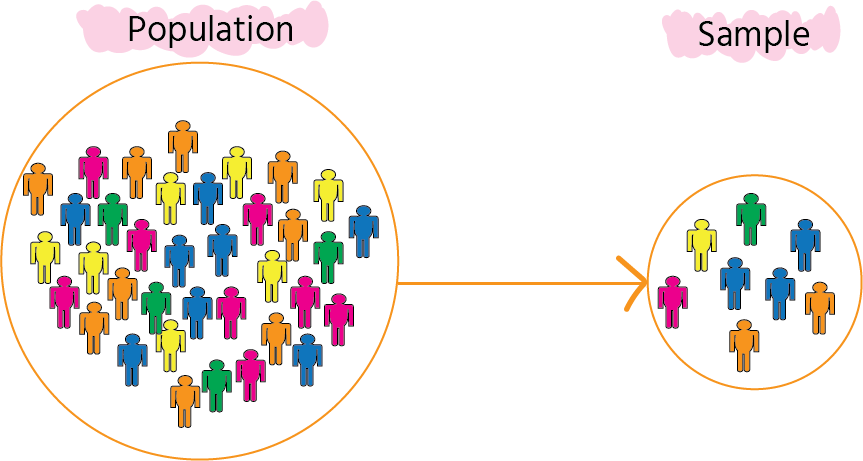Course Content

# Learning Statistics with Python

Learning Statistics with Python

##Sample vs Population

Understanding statistics will expand your horizons and allow you to recognize statistical patterns and work with data. Let's begin with the fundamental definitions: sample and population.Sample

A sample is an unbiased subset of the population that captures the characteristics of the population.

Population

The population is a set of identical items in our study of interest.

Sample vs Population: Example

 Population Sample Example: All the students in the class Top 5 students in the class Example: All students graduated from university 100 random students graduated from university